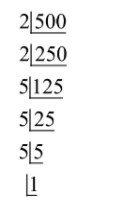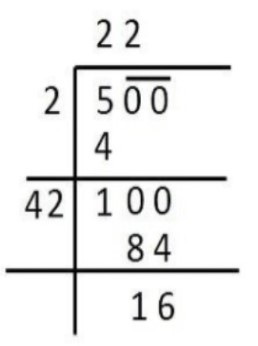There are 500 children in a school. For a P.T. drill they have to stand in such a manner that the number of rows is equal to the number of columns. How many children would be left out in this arrangement?Verified
79.3k+ views
Hint:Given that students are standing in such a manner that number of rows and number of columns are equal in a P.T. drill. Then, first break the number (of students) as the product of its factors and then make pairs of numbers. The numbers left in the product whose pairs are not possible, will be the number of students left out in this arrangement.

Number of students in the school = 500
500 can be written asi.e., $500 = 2 \times 2 \times 5 \times 5 \times 5$
$\Rightarrow \sqrt {500} = \sqrt {2 \times 2 \times 5 \times 5 \times 5} = 10\sqrt 5 = 10 \times 2.03 = 22.03 \approx 22$
$\Rightarrow$In each row or each column maximum 22 students can be arranged
$\Rightarrow$maximum students can participate in P.T. drill will ${\left( {22} \right)^2}$
Therefore, the number of students left out$= 500 - {\left( {22} \right)^2} = 500 - 484 = 16$.

Note:The question can also be done by assuming that
Number of students in rows be x and number of students in columns are in the same number x.
Then, $x \times x = 500 \Rightarrow x = \sqrt {500}$Here the remainder is 16, which is the number of students left out.
The H.C.F. defines the greatest factor present in between given two or more numbers, whereas L.C.M. defines the least number which is exactly divisible by two or more numbers. H.C.F. is also called the greatest common factor (GCF) and LCM is also called the Least Common Divisor.